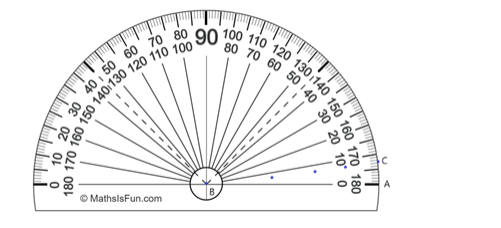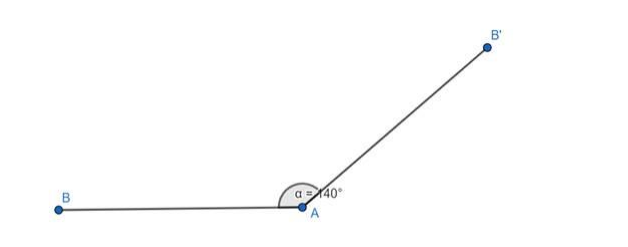# Using a protractor, draw each of the following angles.

Question:

Using a protractor, draw each of the following angles.

$-220^{\circ}$

Solution:

Given angle can be completely written in degree as $=-220^{\circ}$$-220^{\circ}=360^{\circ}-220^{\circ}=140^{\circ}$